#### Thank you for registering.

One of our academic counsellors will contact you within 1 working day.

Please check your email for login details.

Click to Chat

1800-1023-196

+91-120-4616500

CART 0

• 0
MY CART (5)

Use Coupon: CART20 and get 20% off on all online Study Material

ITEM
DETAILS
MRP
DISCOUNT
FINAL PRICE
Total Price: Rs.

There are no items in this cart.
Continue Shopping• Complete JEE Main/Advanced Course and Test Series
• Offered Price: Rs. 15,900
• View Details

# Chapter 17: Symmetry Exercise 17.3

### Question: 1

Complete the following table:

 Shapes Rough figure Number of lines of symmetry (i) scalene triangle0 (ii) Isosceles triangle1 (iii) equilateral triangle (iv) Rectangle (v) Parallelogram (vii) Rhombus (viii) Line (ix) Line segment (x) Angle (xi) Isosceles trapezium (xii) Kite (xiii) Arrow head (xiv) Semi – circle (xv) Circle (xvi) Regular pentagon (xvii) Regular hexagon

### Solution:

 Shapes Rough figure Number of lines of symmetry (i) scalene triangle0 (ii) Isosceles triangle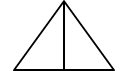1 (iii) equilateral triangle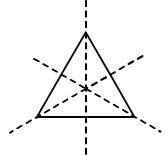3 (iv) Rectangle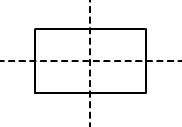4 (v) Parallelogram0 (vii) Rhombus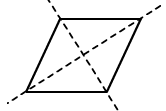2 (viii) Line InfinitelyMany (ix) Line segment1 (x) Angle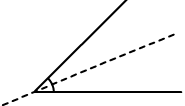1 (xi) Isosceles trapezium1 (xii) Kite1 (xiii) Arrow head1 (xiv) Semi – circle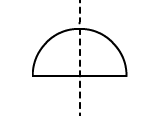1 (xv) Circle Infinitely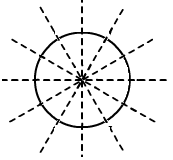Many (xvi) Regular pentagon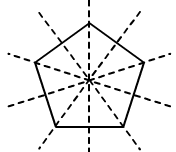5 (xvii) Regular hexagon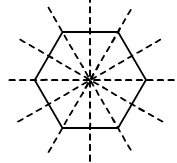6

### Question: 2

Consider the English alphabets A to Z. List among them the letters which have

(i)Vertical line of symmetry

(ii)Horizontal line of symmetry

(iii)Vertical and Horizontal line of symmetry

(iv)No line of symmetry

### Solution:

(i) Vertical line of symmetry:(ii) Horizontal line of symmetry: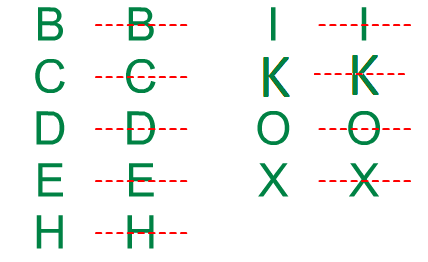(iii) Vertical and Horizontal line of symmetry:(iv) No line of symmetry:### Question: 3

No line of symmetry?

(i) Exactly one line of symmetry.

(ii) Exactly two line of symmetry.

(iii) Three line of symmetry.

(iv) no lines of symmetry

### Solution:

(i) Exactly one line of symmetry:

Yes; isosceles triangle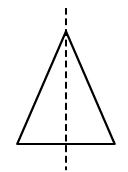(ii) Exactly two line of symmetry:

No

(iii) Three line of symmetry:

Yes; equilateral triangle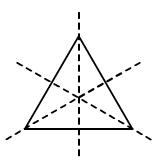(iv) no lines of symmetry

Yes; scalene triangle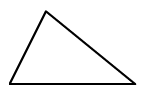### Question: 4

On a squared paper, sketch the following:

(i) A triangle with a horizontal with both horizontal and vertical line of symmetry

(ii) A quadrilateral with both horizontal and vertical lines of symmetry

(iii) A quadrilateral with horizontal but no vertical lines of symmetry

(iv) A hexagon with exactly two lines of symmetry

(v) A hexagon with exactly six lines of symmetry

### Solution: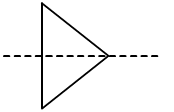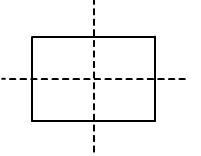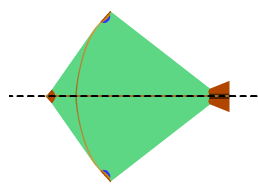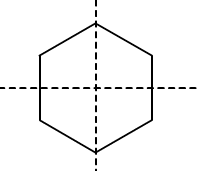(i) (ii) (iii) (iv) (v)

### Question: 5

Draw neat diagrams showing the line (or lines) of symmetry and give the specific name to the quadrilateral having:

(i) only one line of symmetry. How many such quadrilaterals are there?

(ii) its diagonals as the only lines of symmetry

(iii) two lines of symmetry other than diagonals

(iv) More than two lines of symmetry

### Solution:

(i) only one line of symmetry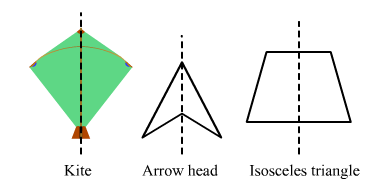(ii) diagonals as the only lines of symmetry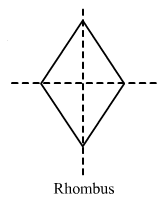(iii) two lines of symmetry other than diagonals:(iv) More than two lines of symmetry:

### Question: 6

write the specific names of all the three quadrilaterals which have only one line of symmetry

### Solution: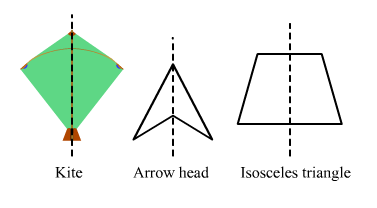### Question: 7

Trace each of the following figures and draw the lines of symmetry. If any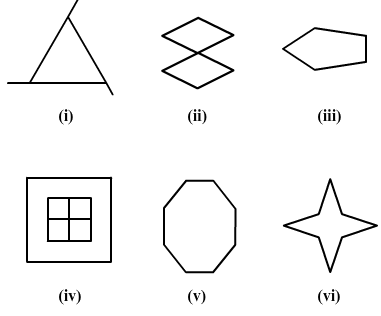### Solution: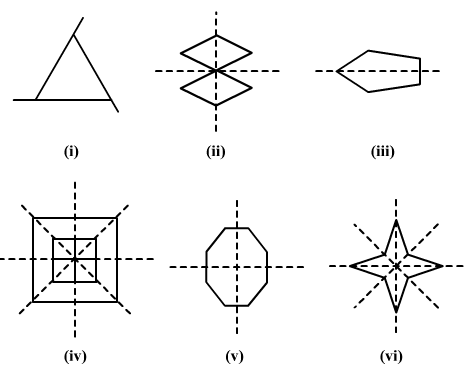### Question: 8

On squared paper copy the triangle in each of the following figures. In each case draw the line(s) of symmetry if any and identify the type of the triangle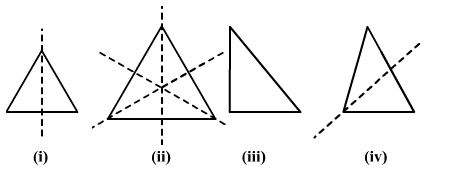### Solution:

(i) This is an isosceles triangle because it has only one line of symmetry.

(ii) This is an Equilateral triangle because it has three lines of symmetry.

(iii) This is a right angled triangle because it has no line of symmetry.

(iv) This is an isosceles triangle it has one line of symmetry.

### Question: 9

Find the lines of symmetry for each of the following shapes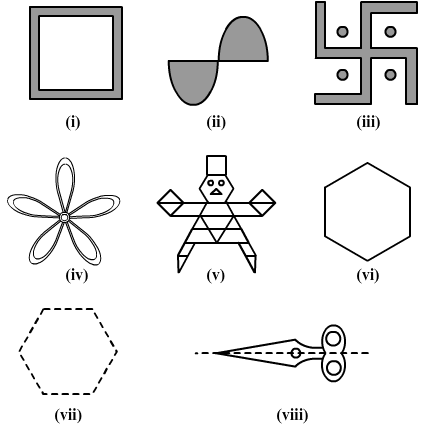### Solution: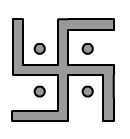(i) (ii) (iii) (iv)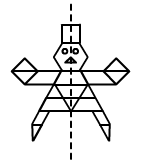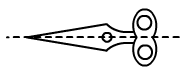(v) (vi) (vii) (viii)

### Question: 10

State whether the following statements are true or false:

(i) A right- angled triangle can have at most two lines of symmetry

(ii) An isosceles triangle with more than one line of symmetry must be an equilateral triangle

(iii) A pentagon with one line of symmetry can be drawn.

(iv) A pentagon with more than one line of symmetry must be regular

(v) A hexagon with one line of symmetry can be drawn

(vi) A hexagon with more than one line of symmetry must be regular

### Solution:

(i)True

If it is an isosceles right angle triangle, then it can have only one line of symmetry at the most. Otherwise, a right angle triangle has no line of symmetry.(ii) If an isosceles triangle has no more than one line of symmetry, then it must be an equilateral triangle. This is because an equilateral triangle has three lines of symmetry, and a triangle other than that cannot have two lines of symmetry.(iii)True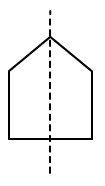(iv)True(v)True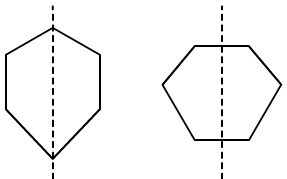(vi)True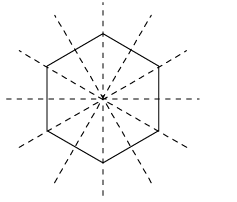## Complete JEE Main/Advanced Course and Test Series

OFFERED PRICE: Rs. 15,900
View Details Xpress Buy

### Course Features

• 731 Video Lectures
• Revision Notes
• Test paper with Video Solution
• Mind Map
• Study Planner
• NCERT Solutions
• Discussion Forum
• Previous Year Papers

### Course Features

• 728 Video Lectures
• Revision Notes
• Previous Year Papers
• Mind Map
• Study Planner
• NCERT Solutions
• Discussion Forum
• Test paper with Video Solution